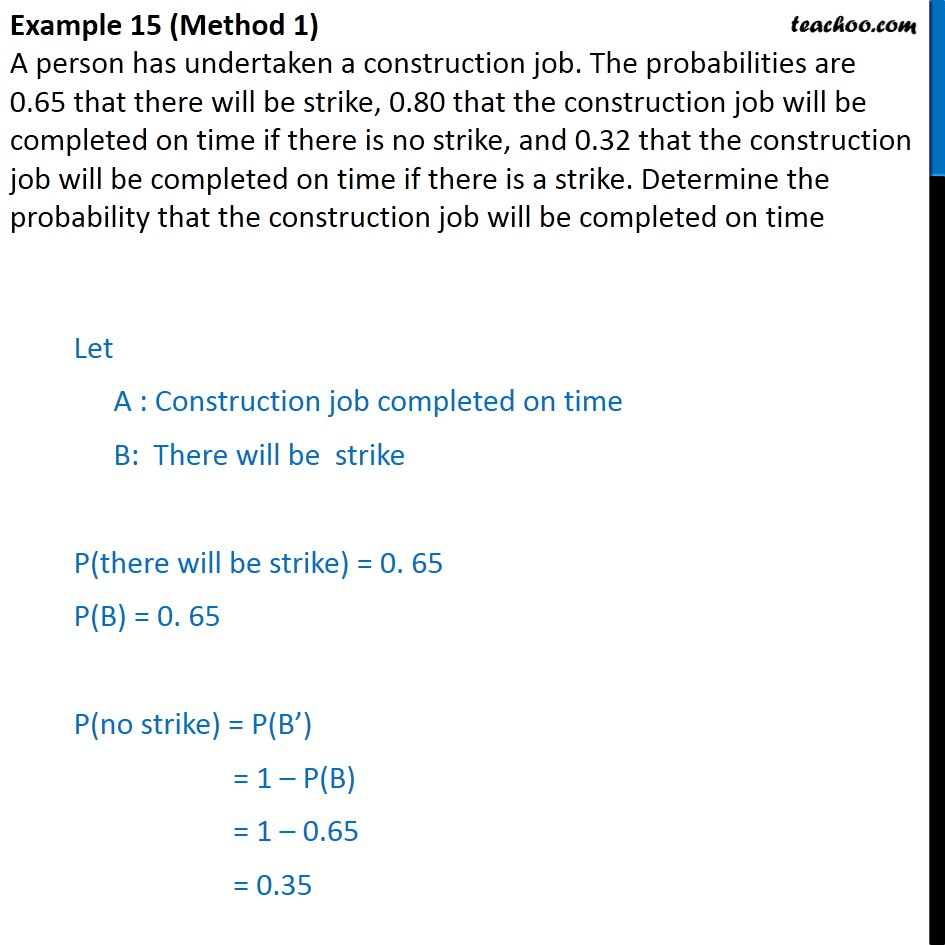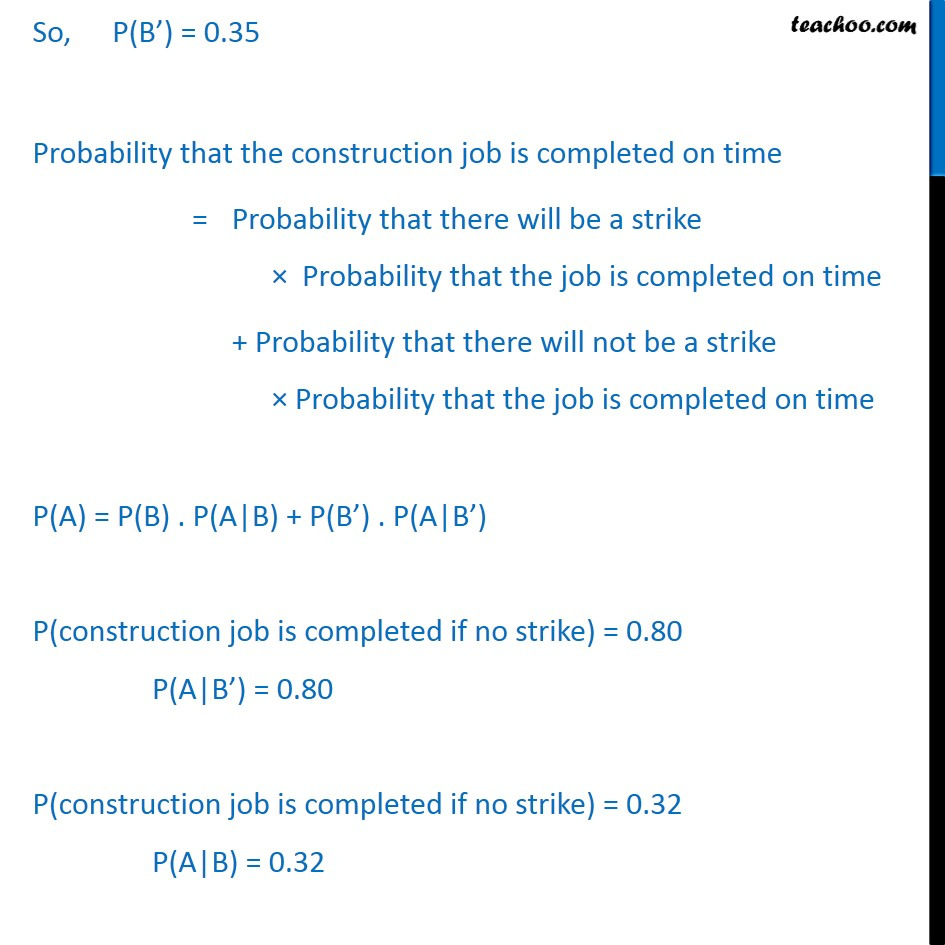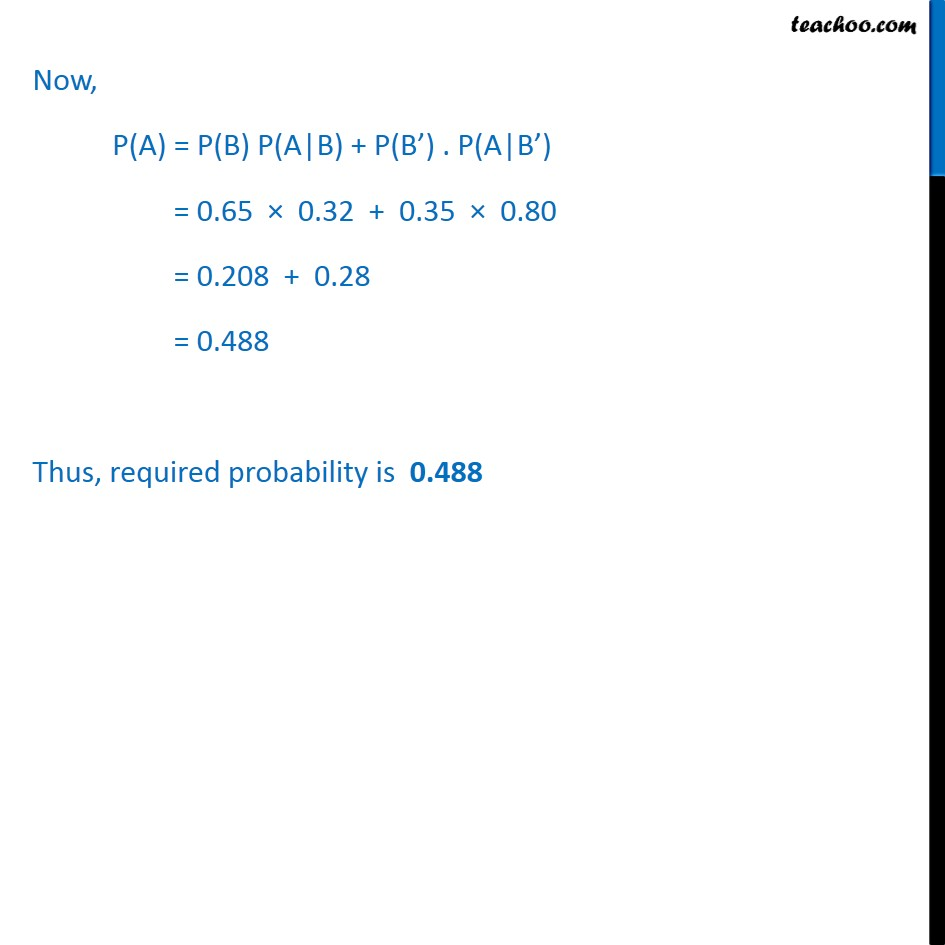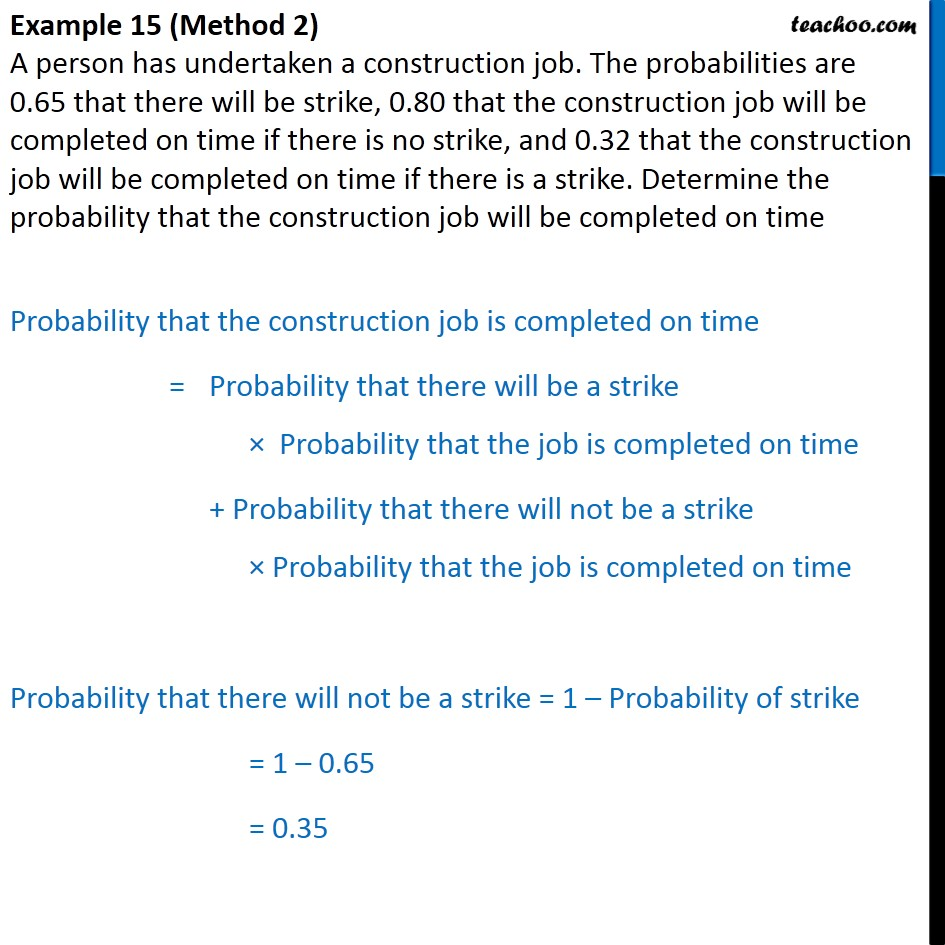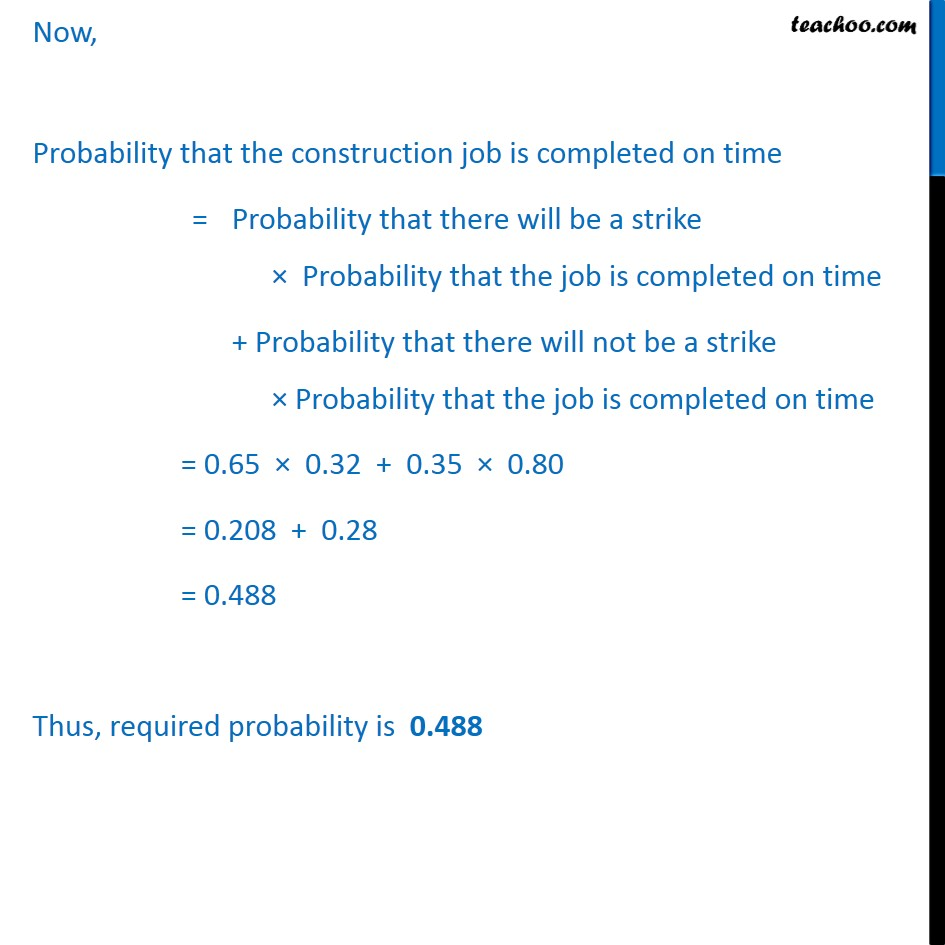1. Chapter 13 Class 12 Probability
2. Serial order wise
3. Examples

Transcript

Example 15 (Method 1) A person has undertaken a construction job. The probabilities are 0.65 that there will be strike, 0.80 that the construction job will be completed on time if there is no strike, and 0.32 that the construction job will be completed on time if there is a strike. Determine the probability that the construction job will be completed on time Let A : Construction job completed on time B: There will be strike P(there will be strike) = 0. 65 P(B) = 0. 65 P(no strike) = P(B’) = 1 – P(B) = 1 – 0.65 = 0.35 So, P(B’) = 0.35 Probability that the construction job is completed on time = Probability that there will be a strike × Probability that the job is completed on time + Probability that there will not be a strike × Probability that the job is completed on time P(A) = P(B) . P(A|B) + P(B’) . P(A|B’) P(construction job is completed if no strike) = 0.80 P(A|B’) = 0.80 P(construction job is completed if no strike) = 0.32 P(A|B) = 0.32 Now, P(A) = P(B) P(A|B) + P(B’) . P(A|B’) = 0.65 × 0.32 + 0.35 × 0.80 = 0.208 + 0.28 = 0.488 Thus, required probability is 0.488 Example 15 (Method 2) A person has undertaken a construction job. The probabilities are 0.65 that there will be strike, 0.80 that the construction job will be completed on time if there is no strike, and 0.32 that the construction job will be completed on time if there is a strike. Determine the probability that the construction job will be completed on time Probability that the construction job is completed on time = Probability that there will be a strike × Probability that the job is completed on time + Probability that there will not be a strike × Probability that the job is completed on time Probability that there will not be a strike = 1 – Probability of strike = 1 – 0.65 = 0.35 Now, Probability that the construction job is completed on time = Probability that there will be a strike × Probability that the job is completed on time + Probability that there will not be a strike × Probability that the job is completed on time = 0.65 × 0.32 + 0.35 × 0.80 = 0.208 + 0.28 = 0.488 Thus, required probability is 0.488

Examples